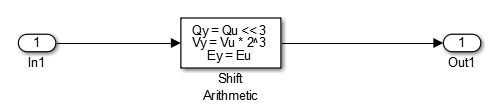Replace Multiplication by Powers of Two with Signed Bitwise Shifts

This example shows how to generate code that replaces multiplication by powers of two with signed bitwise shifts. Code that contains bitwise shifts executes faster than the code containing multiplication by powers of two.

Some coding standards, such as MISRA, do not allow bitwise operations on signed integers. If you want to increase your chances of producing MISRA C compliant code, do not replace multiplication by powers of two with bitwise shifts.

Example

To replace multiplication by powers of two with bitwise shifts, create the following model. In this model, a signal of Data type int16 feeds into a Shift Arithmetic block. In the Shift Arithmetic Block Parameters dialog box, the Bits to shift > Direction parameter is set to Left. The Bits to shift > Number parameter is set to 3. This parameter corresponds to a value of 8, or raising 2 to the power of 3.Generate Code with Signed Bitwise Shifts

1. Open the Model Configuration Parameters dialog box and select the Code Style tab. The Replace multiplications by powers of two with signed bitwise shifts parameter is on by default.

2. Generate code for the model.

In the bitwise_multiplication.c file, the bitwise_multiplication step function contains this code:

bitwise_multiplication_Y.Out1 = (int16_T)(bitwise_multiplication_U.In1 << 3);

The signed integer, bitwise_multiplication_U.In1, is shifted three bits to the left.

Generate Code with Multiplication by Powers of Two

1. Open the Model Configuration Parameters dialog box and select the Code Style tab.

2. Clear the Replace multiplications by powers of two with signed bitwise shifts parameter.

3. Generate code for the model.

In the bitwise_multiplication.c file, the bitwise_multiplication step function contains this code:

bitwise_multiplication_Y.Out1 = (int16_T)(bitwise_multiplication_U.In1 * 8);

The signed integer bitwise_multiplication_U.In1 is multiplied by 8.